• ## 导入mnist数据集

千次阅读 2019-04-07 19:56:16
下载一个代码后，发现需要导入mnist数据集，首先新建一个py的文件，把代码复制过来，然后记得一定要改成这样的格式： from tensorflow.examples.tutorials.mnist import input_data mnist = input_data.read_data_...
下载一个代码后，发现需要导入mnist数据集，首先新建一个py的文件，把代码复制过来，然后记得一定要改成这样的格式：
from tensorflow.examples.tutorials.mnist import input_data

这代表：read_data_sets()的作用就是检查目录下有没有想要的数据，没有的话下载，然后进行解压、 在工作目录中新建一个MNIST_data文件夹，用于存放下载的数据集，新建好后不要放任何文件！任何运行代码就行了，他可以自己进行下载数据集解压到这个文件中。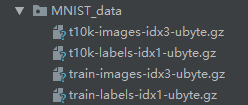解压后效果，有四个文件。 CNN训练mnist数据集代码如下：手写数字，代码来自上一篇博客。就改了本文刚开始写的那段代码！
  #!/usr/bin/env python
# -*- coding: utf-8 -*-

import tensorflow as tf

# 加载测试数据的读写工具包，加载测试手写数据，目录MNIST_data是用来存放下载网络上的训练和测试数据的。
# 这里，参考我前面的博文，由于网络原因，测试数据，我单独下载后，放在当前目录的MNIST_data目录了。
import tensorflow.examples.tutorials.mnist.input_data as input_data

# 创建一个交互式的Session。
sess = tf.InteractiveSession()

# 创建两个占位符，数据类型是float。x占位符的形状是[None，784]，即用来存放图像数据的变量，图像有多少张
# 是不关注的。但是图像的数据维度有784围。怎么来的，因为MNIST处理的图片都是28*28的大小，将一个二维图像
# 展平后，放入一个长度为784的数组中。
# y_占位符的形状类似x，只是维度只有10，因为输出结果是0-9的数字，所以只有10种结构。
x = tf.placeholder("float", shape=[None, 784])
y_ = tf.placeholder("float", shape=[None, 10])

# 通过函数的形式定义权重变量。变量的初始值，来自于截取正态分布中的数据。
def weight_variable(shape):
initial = tf.truncated_normal(shape, stddev=0.1)
return tf.Variable(initial)

# 通过函数的形式定义偏置量变量，偏置的初始值都是0.1，形状由shape定义。
def bias_variable(shape):
initial = tf.constant(0.1, shape=shape)
return tf.Variable(initial)

# 定义卷积函数，其中x是输入，W是权重，也可以理解成卷积核，strides表示步长，或者说是滑动速率，包含长宽方向
# 际卷积中，参与计算的原始图像数据都会参与。一种是VALID，补齐操作后，进行卷积过程中，原始图片中右边或者底部
# 的像素数据可能出现丢弃的情况。
def conv2d(x, w):
return tf.nn.conv2d(x, w, strides=[1, 1, 1, 1], padding='SAME')

# 这步定义函数进行池化操作，在卷积运算中，是一种数据下采样的操作，降低数据量，聚类数据的有效手段。常见的
# 池化操作包含最大值池化和均值池化。这里的2*2池化，就是每4个值中取一个，池化操作的数据区域边缘不重叠。
# 函数原型：def max_pool(value, ksize, strides, padding, data_format="NHWC", name=None)。对ksize和strides
# 定义的理解要基于data_format进行。默认NHWC，表示4维数据，[batch,height,width,channels]. 下面函数中的ksize，
# strides中，每次处理都是一张图片，对应的处理数据是一个通道（例如，只是黑白图片）。长宽都是2，表明是2*2的
# 池化区域，也反应出下采样的速度。
def max_pool_2x2(x):
return tf.nn.max_pool(x, ksize=[1, 2, 2, 1], strides=[1, 2, 2, 1], padding='SAME')

# 定义第一层卷积核。shape在这里，对应卷积核filter。
# 其中filter的结构为：[filter_height, filter_width, in_channels, out_channels]。这里，卷积核的高和宽都是5，
# 输入通道1，输出通道数为32，也就是说，有32个卷积核参与卷积。
W_conv1 = weight_variable([5, 5, 1, 32])
# 偏置量定义，偏置的维度是32.
b_conv1 = bias_variable()

# 将输入tensor进行形状调整，调整成为一个28*28的图片，因为输入的时候x是一个[None,784]，有与reshape的输入项shape
# 是[-1,28,28,1]，后续三个维度数据28,28,1相乘后得到784，所以，-1值在reshape函数中的特殊含义就可以映射程None。即
# 输入图片的数量batch。
x_image = tf.reshape(x, [-1,28,28,1])

# 将2维卷积的值加上一个偏置后的tensor，进行relu操作，一种激活函数，关于激活函数，有很多内容需要研究，在此不表。
h_conv1 = tf.nn.relu(conv2d(x_image, W_conv1) + b_conv1)
# 对激活函数返回结果进行下采样池化操作。
h_pool1 = max_pool_2x2(h_conv1)

# 第二层卷积，卷积核大小5*5，输入通道有32个，输出通道有64个，从输出通道数看，第二层的卷积单元有64个。
W_conv2 = weight_variable([5, 5, 32, 64])
b_conv2 = bias_variable()

# 第二层卷积：激活和池化（类似第一层卷积操作的激活和池化）
h_conv2 = tf.nn.relu(conv2d(h_pool1, W_conv2) + b_conv2)
h_pool2 = max_pool_2x2(h_conv2)

# 图片尺寸减小到7x7，加入一个有1024个神经元的全连接层，用于处理整个图片。把池化层输出的张量reshape成一些
# 向量，乘上权重矩阵，加上偏置，然后对其使用ReLU激活操作。
W_fc1 = weight_variable([7 * 7 * 64, 1024])
b_fc1 = bias_variable()

# 将第二层池化后的数据进行变形
h_pool2_flat = tf.reshape(h_pool2, [-1, 7*7*64])
# 进行矩阵乘，加偏置后进行relu激活
h_fc1 = tf.nn.relu(tf.matmul(h_pool2_flat, W_fc1) + b_fc1)

keep_prob = tf.placeholder("float")
# 对第二层卷积经过relu后的结果，基于tensor值keep_prob进行保留或者丢弃相关维度上的数据。这个是为了防止过拟合，快速收敛。
h_fc1_drop = tf.nn.dropout(h_fc1, keep_prob)

W_fc2 = weight_variable([1024, 10])
b_fc2 = bias_variable()

# 最后，添加一个softmax层，就像前面的单层softmax regression一样。softmax是一个多选择分类函数，其作用和sigmoid这个2值
# 分类作用地位一样，在我们这个例子里面，softmax输出是10个。
y_conv=tf.nn.softmax(tf.matmul(h_fc1_drop, W_fc2) + b_fc2)

# 实际值y_与预测值y_conv的自然对数求乘积，在对应的维度上上求和，该值作为梯度下降法的输入
cross_entropy = -tf.reduce_sum(y_*tf.log(y_conv))

# 首先分别在训练值y_conv以及实际标签值y_的第一个轴向取最大值，比较是否相等
correct_prediction = tf.equal(tf.argmax(y_conv,1), tf.argmax(y_,1))

# 对correct_prediction值进行浮点化转换，然后求均值，得到精度。
accuracy = tf.reduce_mean(tf.cast(correct_prediction, "float"))

# 先通过tf执行全局变量的初始化，然后启用session运行图。
sess.run(tf.global_variables_initializer())
for i in range(20000):
# 从mnist的train数据集中取出50批数据，返回的batch其实是一个列表，元素0表示图像数据，元素1表示标签值
batch = mnist.train.next_batch(50)
if i % 100 == 0:
# 计算精度，通过所取的batch中的图像数据以及标签值还有dropout参数，带入到accuracy定义时所涉及到的相关变量中，进行
# session的运算，得到一个输出，也就是通过已知的训练图片数据和标签值进行似然估计，然后基于梯度下降，进行权值训练。
train_accuracy = accuracy.eval(feed_dict={x:batch, y_: batch, keep_prob: 1.0})
print("step %d, training accuracy %g"%(i, train_accuracy))
# 此步主要是用来训练W和bias用的。基于似然估计函数进行梯度下降，收敛后，就等于W和bias都训练好了。
train_step.run(feed_dict={x: batch, y_: batch, keep_prob: 0.5})

# 对测试图片和测试标签值以及给定的keep_prob进行feed操作，进行计算求出识别率。就相当于前面训练好的W和bias作为已知参数。
print("cf accuracy %g"%accuracy.eval(feed_dict={x: mnist.test.images, y_: mnist.test.labels, keep_prob: 1.0}))

测试效果如下：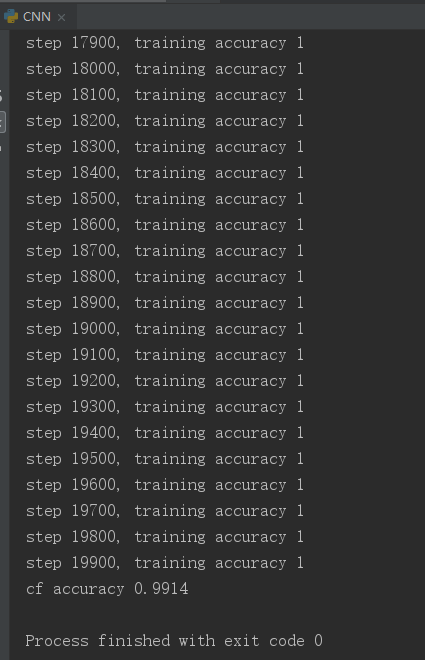展开全文• 1.一开始采用官网上利用input_data来...2.后面采用 keras加载mnist数据集，又会报出如下错误 URL fetch fail:http://googelsourc…………………………………… 解决办法：先将mnist数据集下载到本地，放到自己想...
注意：使用keras模块加载mnist数据集时，可以不用修改代码，直接将mnist数据集放到相应的路径下面。
windows: C:\Users\mac\.keras\datasets

1.一开始采用官网上利用input_data来加载本地数据集的方法，但会报出下面的错误
No module named 'tensorflow.examples.tutorials'

并且官网上input_data.py又下载不下来 2.采用keras，一开始也是因为无法访问googlesource,导致无法加载mnist数据集。 解决方法：修改mnist.py（使用 ctrl + b，打开mnist.py），利用本地下载好的mnist数据集，直接将mnist.py里路径path改成本地mnist数据集的路径 下附代码： main.py
from __future__ import absolute_import, division, print_function, unicode_literals
import tensorflow as tf

mnist = tf.keras.datasets.mnist

(x_train, y_train), (x_test, y_test) = mnist.load_data()
x_train, x_test = x_train / 255.0, x_test / 255.0

model = tf.keras.models.Sequential([
tf.keras.layers.Flatten(input_shape=(28, 28)),
tf.keras.layers.Dense(128, activation='relu'),
tf.keras.layers.Dropout(0.2),
tf.keras.layers.Dense(10, activation='softmax')
])

loss='sparse_categorical_crossentropy',
metrics=['accuracy'])

model.fit(x_train, y_train, epochs=5)

model.evaluate(x_test,  y_test, verbose=2)


mnist.py

"""MNIST handwritten digits dataset.
"""
from __future__ import absolute_import
from __future__ import division
from __future__ import print_function

import numpy as np

from tensorflow.python.keras.utils.data_utils import get_file
from tensorflow.python.util.tf_export import keras_export

Arguments:
path: path where to cache the dataset locally
(relative to ~/.keras/datasets).

Returns:
Tuple of Numpy arrays: (x_train, y_train), (x_test, y_test).

Yann LeCun and Corinna Cortes hold the copyright of MNIST dataset,
which is a derivative work from original NIST datasets.
MNIST dataset is made available under the terms of the
"""

path = "./mnist.npz"
x_train, y_train = f['x_train'], f['y_train']
x_test, y_test = f['x_test'], f['y_test']

return (x_train, y_train), (x_test, y_test)

展开全文数据集
• 解决pytorch本地导入mnist数据集报错问题


目录
问题描述：解决方法：

问题描述：
众所周知国内的网络的问题，mnist(MNIST, FashionMNIST, EMNIST)数据集不搭梯子现在是不能自动下载了。
当我去网上下载好mnist数据集之后，据说要放在：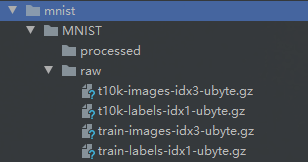然后我的代码是这样的：
import torch
from torch import nn, optim
from torchvision import transforms, datasets

import visdom

def main():
mnist_train = datasets.MNIST(root='./mnist', train=True, transform=transforms.Compose([
transforms.ToTensor()

mnist_test = datasets.MNIST(root='./mnist', train=False, transform=transforms.Compose([
transforms.ToTensor()
x, _ = iter(mnist_train).next()
print('x:', x.shape)

if __name__ == '__main__':
main()


运行之后是这样的：
Dataset not found. You can use download=True to download it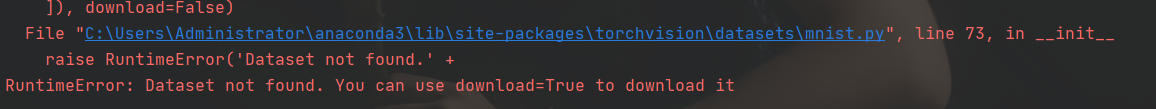root='./mnist这个路径改了又改也不行
最后发现是缺少俩文件：解决方法：
github上一个大佬提供了EMNIST全系列的.pt文件
链接：torchvision-datasets-mnist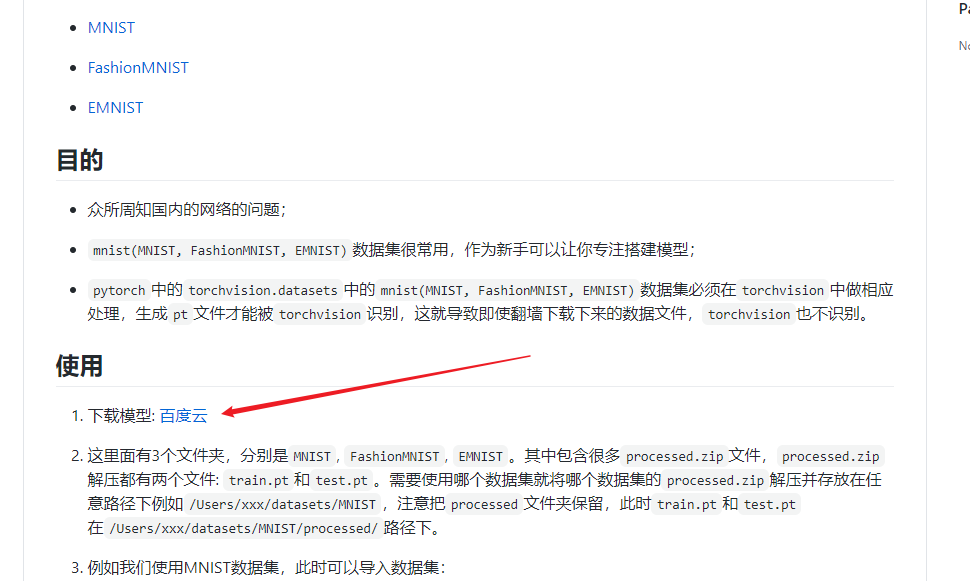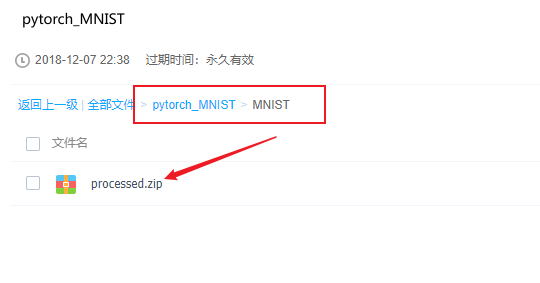最后的目录（代码没改，路径还是./mnist）：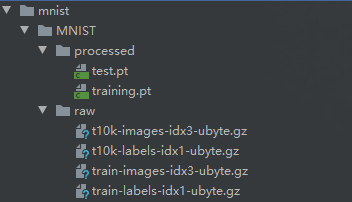运行完美：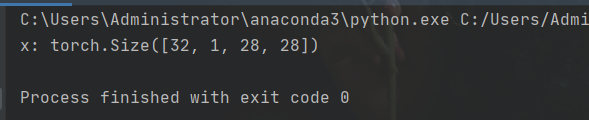如果大佬的链接失效了，那我给出我的（只有mnist）：
链接：https://pan.baidu.com/s/1f7Ok62Gg2xcnz4ZpQiVXiw 提取码：jdzl 复制这段内容后打开百度网盘手机App，操作更方便哦–来自百度网盘超级会员V5的分享
展开全文• TensorFlow 2.0 快速导入MNIST数据集 由于当初下载的Tensorflow是2.0版本的与《智能通信：基于深度学习的物理层设计》一书的版本不兼容，在第三章中导入MNIST数据中出现问题。 在Tensorflow1.0中导入数据库用如下...
TensorFlow 2.0 快速导入MNIST数据集
由于当初下载的Tensorflow是2.0版本的与《智能通信：基于深度学习的物理层设计》一书的版本不兼容，在第三章中导入MNIST数据中出现问题。
在Tensorflow1.0中导入数据库用如下代码由于版本不兼容，出现波浪线错误在Tensorflow2.0中可以用如下代码导入MNIST数据集结果如下`
展开全文tensorflow
• 如何导入mnist数据集并且预处理 mnist是我们在学习机器学习或者深度学习时常用的数据集，以下是使tensorflow导入mnist的一种方法。 在深度学习训练中，为了提高执行效率，常进行矩阵操作，因此后面还对数据集进行的...tensorflow 深度学习
• Pycharm tensorflow2.3.1无法导入mnist数据集，程序运行报错No module named tensorflow.examples.tutorials.mnist from tensorflow.examples.tutorials.mnist import input_data mnist = input_data.read_data_sets...tensorflow
• sklearn学习过程中无法导入MNIST数据集 今天在学习SVM识别MNIST数据集的过程中，代码运行一直报错，无法连接到主机，也就是无法下载MNIST数据集。网上查了好多中方法，对我这种新手来说都有点费力看懂，导致解决了...python
• 按照TensorFlow官方文档导入MNIST数据集失败的解决TensorFlow
• ImportError: DLL load failed with error code -1073741795tensorflow 导入mnist数据集是出现无法导入的错误 搜索了很多相关问题终于找到了答案，这位同志也遇到了相同的问题，楼下有人给出了我需要的答案 由于...
• 首先下载： 下载链接 下载右边4个文件，并存在新建的MNIST_data文件夹中。注意⚠️千万不要解压！否则就会不停报错超时！...导入mnist数据集： import tensorflow as tf from tensorflow.examples.tutorials.mnist...python
• 一、MNIST数据集介绍 首先你需要从官网上下载mnist相应文件，一共四个文件。其中训练数据集包含60000个数字图片，测试数据集包含10000个图片用作检测使用，下图是相应文件及其相应文件存储方式。 TRAINING SET ...Python
• ## Keras导入Mnist数据集出错解决方案

千次阅读 多人点赞 2018-10-31 09:57:07
Mnist数据集导入出错 在进行Mnist手写识别的项目中，出现了Mnist数据集下载出错的问题，报出以下错误： Exception: URL fetch failure on https://s3.amazonaws.com/img-datasets/mnist.npz: None -- [WinError ...卷积神经网络 深度学习
• 机器学习实战（从本地导入mnist数据集） 第三章这里总是报错，从本地导入好久也没有成功，在网上也搜索了好几天，成功了没有记录下来，在第八章降维中又用到了数据集，查了一下午，终于找到了解决方案，所以记录...机器学习
• 问题 当我使用github上别人的代码： ...from tensorflow.examples.tutorials.mnist import input_data from tensorflow.examples.tutorials.mnist import input_data mnist = input_data.read_data_sets("/t...tensorflow python
• #mnist = input_data.read_data_sets('MNIST_data', one_hot=True) from tensorflow.examples.tutorials.mnist import input_data mnist = input_data.read_data_sets("MNIST_data/", one_hot=True) 转载于:...
• from tensorflow.examples...., one_hot=True)这是官方文档给出的导入代码，但我同很多人有一样的问题，运行程序的时候发现并没有讲MNIST数据集导入，so，在网上找了很多资料，最后找到了解决方法。先在你正在...tensorflow
• 在利用Tensorflow学习深度学习的过程中，相信很多人第一次写的代码就是利用mnist数据集进行手写数字识别。这个例子足够经典，能够让我们对Tensorflow的使用，比如创建占位符、Softmax回归、利用简单代码实现模型、...Tensorflow 网络错误
• 项目场景：TensorFlow2.4在使用MNIST数据集 在使用MNIST数据集时，载入数据集与使用函数时的问题 问题描述： 使用“from tensorflow.examples.tutorials.mnist import input_data”报错 运行时出现“No module named...tensorflow 深度学习
• 当我运行下面代码时，提示我无法下载mnist数据集。 mnist = input_data.read_data_sets("data", one_hot=True) # 设置变量 x = tf.placeholder("float", [None, 784]) W = tf.Variable(tf.......